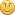Started by Hetzen, September 30, 2019, 08:43:45 AM

#### Hetzen

Add Multiplied Scalar will multiple the Main Input with Input 2 and add that result with the Main Input.

Notice this node only looks at Scalar information and ignores any displacement. If the input values are positive, this node will essentially masks Input 2 and add that to the Main Input as you can see in following example.If Input 2 is disconnected, the Main Input passes through to the Output.If the Main Input is disconnected, nothing passes through.This node is a compact way of using an Add Scalar and Multiply Scalar connected in the below example, and can be useful in combining different scales of noise.#### WAS

#1
I would like you to expand on this, and clarify some information, please.No input is masked. It's multiplied by "black" or 0. The data is still there, especially when worked with unclamped scalars. It's been essentially floored to 0. Additionally, negative unclamped values will be multiplied, as well as values above 1.

#### Hetzen

#2
Thanks Jordan, well spotted. I've corrected the first post to say positive values re-masking.

#### WAS

#3
Well is information being masked or is it multiplied? Sure it can be used as a mask.

What about the scalar data you are trying to represent? Its chief use is displacement maps (otherwise might as well use Colour functions).

So what about what's actually going on?

What's happening to the data in formula that's representative of mathematics? For example, what about when two locations in both maps contain a value of 0.5 where another has 1, or where both have a value of 1 (or above) and add multiplied? What is the scalar value which would be displacement amplitude?

This post is sorta very basic and just visual, and doesn't correctly cover the function.

#### WAS

#4
These are all mainly mathematical functions, and I think that's the main focal area that should be being explained. What's happening with these functions under the hood. Visual results are important, but not that important to what's being done inside, or what the result actually translates too in data.

#### Hetzen

#5
Add Multiplied Scalar as in the last image of the first post is a compact way of connecting an Add Scalar with a Multiply Scalar together in the way that's shown. That's all that is going on.

So if you have a value of 0.5 in the Main Input and 1 in Input 2, the maths is:

0.5 x 1 + 0.5 = 1

If the Main Input is -1 and Input 2 is 1:

-1 x 1 + -1 = -2

or with Main Input is -0.5 and Input 2 is 2:

-0.5 x 2 + -0.5 = -1.5

and so on.

If you add vectors or colour into the inputs, there is a conversion that happens to those three component data streams to make a scalar value with one component. I've tried to cover that in Add Scalar and you can find further information in the the wiki here.

Masking (which may have been a descriptive error on my part in the initial post) is essentially multiplying one scalar value with another to hopefully produce an alpha mask of a range of 0 to 1. Black to white.
As you mentioned, you can put in other values which fall out of this range of 0 to 1 depending on the input values.

#### Oshyan

#6
Posts about the goals and process around the "Functions Nodes in Practice" forum area as a whole have been split into their own discussion.
https://planetside.co.uk/forums/index.php/topic,27174.0.html

Please keep the individual node discussion threads focused on the node in question.

- Oshyan

#### Tangled-Universe

#7
(Oshyan please feel free to move this elsewhere as this starts on-topic and progresses into slightly off-topic)

Hi Jon,

I don't mean to nitpick, but if I read "add multiplied scalar" it means to me I'm adding 2 multiplied values to an existing value.
The existing value is input 1 or say 'A'.
The value to multiply and add (notice the swapped notation) is input 2 or say 'B'.
That would be notated like A+(AxB).
Your notation (AxB)+A translates in my brain to "multiply scalar add input".

This may sound ridiculous to you haha, but to me these subtleties make a lot of difference in understanding what's going on.
I'm not sure how to find the right words which describe my thoughts, but I think this notation fits closer TG's nature of treating nodes.
This notation fits the node UI from left to right and to me more intuitively describes what the node does.
I literally visualize the input value on the left and what the input on the right would do to it, so when notation is reversed I have a hard time following.
Your notation is mathematically 100% correct and valid, as there's nothing wrong with swapping operations in this case, but to me it's very counter-intuitive to the description and node interface.
I hope you can see a bit what I mean.
I don't mean to nitpick, my brain just works in a few dimensions lower than yours when it comes to math.

#### Hetzen

#8
Thanks Martin. I've tried to use the names of the inputs otherwise I would agree A and B is better notation. The order of A+(AxB) does read better as well, I guess I was writing it down in the order the calculation happens.